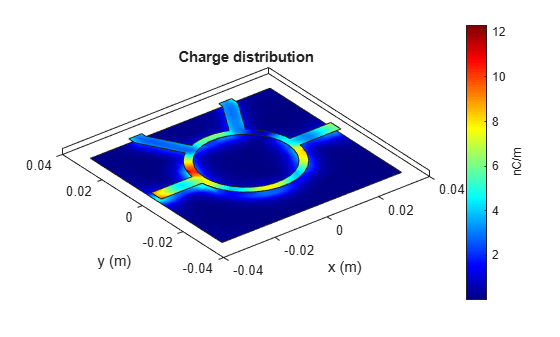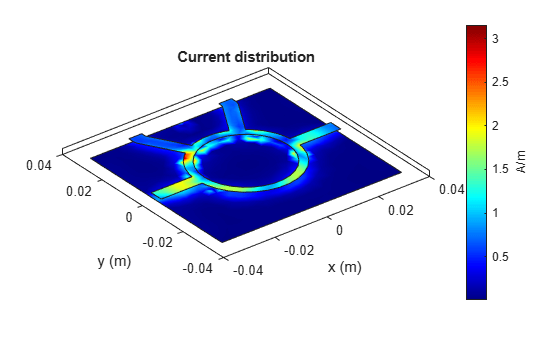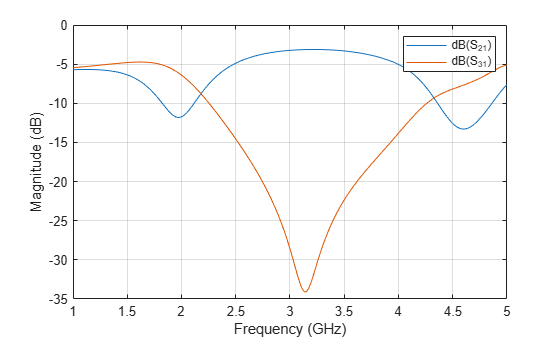# Rat-Race Coupler - Visualize and Analyze

This example shows you how to create, visualize, and analyze a Rat-Race Coupler.

Create a rat-race coupler with default properties.

`ratrace = couplerRatrace`
```ratrace = couplerRatrace with properties: PortLineLength: 0.0186 PortLineWidth: 0.0050 CouplerLineWidth: 0.0030 Circumference: 0.1110 Height: 0.0016 Substrate: [1×1 dielectric] Conductor: [1×1 metal] ```

View the coupler.

`show(ratrace)`Plot the charge distribution at 5 GHz.

`charge(ratrace, 5e9)`Plot the current distribution at 5 GHz.

```figure current(ratrace, 5e9)```Calculate and plot the s-parameters.

`spar = sparameters(ratrace,linspace(1e9,5e9,20))`
```spar = sparameters: S-parameters object NumPorts: 4 Frequencies: [20×1 double] Parameters: [4×4×20 double] Impedance: 50 rfparam(obj,i,j) returns S-parameter Sij ```
`rfplot(spar)````figure rfplot(spar,[2 3],1)```